# Energy in an Inductor

When a electric current is flowing in an inductor, there is energy stored in the magnetic field. Considering a pure inductor L, the instantaneous power which must be supplied to initiate the current in the inductor is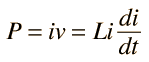so the energy input to build to a final current i is given by the integral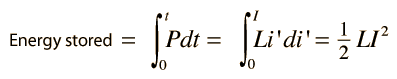Using the example of a solenoid, an expression for the energy density can be obtained.

Index

Inductance concepts

Capacitance concepts

 HyperPhysics***** Electricity and Magnetism R Nave
Go Back

# Energy in Magnetic Field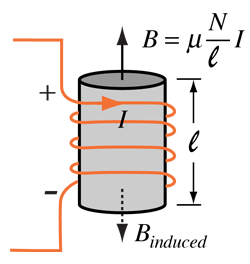Solenoid Field Solenoid Inductance

From analysis of the energy stored in an inductor,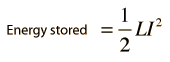the energy density (energy/volume) is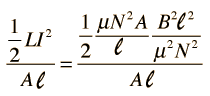so the energy density stored in the magnetic field is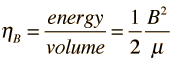Index

Inductance concepts

Capacitance concepts

 HyperPhysics***** Electricity and Magnetism R Nave
Go Back